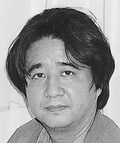MATSUO Atsushi

MATSUO AtsushiTitle Associate Professor Department of Mathematics (UG), Graduate School of Mathematical Sciences561, Graduate School of Mathematical Sciences Bldg. 5F, Komaba Campus +81-3-5465-8346　|　48346（ext.）

## Research Field

Group theory, Representation theory, Mathematical physics

## Research Subject

Mathematical structures related to conformal field theories

## Current Research

The aim of our research is to understand the mathematical structures related to 2-dimensional conformal field theories by looking at the system of axioms for vertex operator algebras. In particular, we have studied the properties of the finite groups which appear as the automorphism groups of vertex operator algebras, and found that there are certain numerical constraints for a vertex operator algebra on which the automorphism group acts in a fixed-point free manner up to some degree.

## Keywords

representation theories, Lie algebras, automorphism group, Monster, moonshine, vertex operator algebras, conformal field theories